# KCSE-Physics

Q1.State five properties of X-rays

• They are not deflected by both electric and magnetic fileds
• They cause ionisation in gases
• They cause photoelectric emission
• They significantly penetrate matter
• They are completely absorbed by lead metalQ2.State five properties  of X-rays

1. They ionises gases
2. They significantly penetrate matter.
3. They affect photographic plates
4. They are completely absorbed by lead metal
5. They are not deflected by both magnetic and electric fileds.Q3.Physics KCSE 2020 Paper 1 Qs 1-2

1. The radius of a wire was measured as 2.43mm. Name the instrument that is used to measures this diameter

Micrometer Screw Gauge

2. Figure 1 shows a capillary tube dipped in mercury in a beaker. State with a reason the observation that would be made on the level of mercury in the capillary tube if the temperature of the mercury is increased

The level rises – cohesive forces become weaker on heating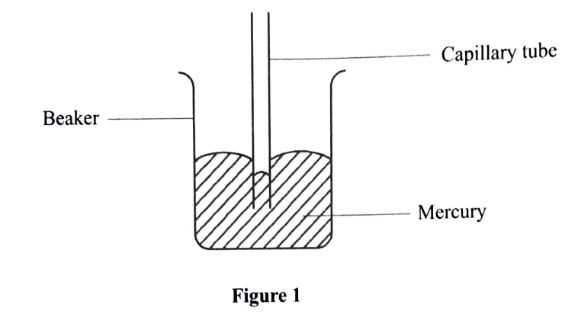Q4.KCSE 2020 Paper 1 Qs. 3-4

3. State the kinetic theory of gases.

It states that gases are made up of tiny molecules that are in constant random motion

4. Figure 2 shows the scale of a measuring instrument.

9.5 Pa

b. State the physical quantity measured in this instrument

Pressure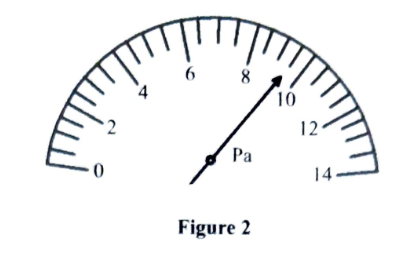Q5.Physics Paper 1 KCSE 2020 Qs. 5

5. Figure 3 shows a traditional stool resting on a level surface.

a.  Identify its state of equilibrium

• Stable equilibrium

• Returns to its original position after displacement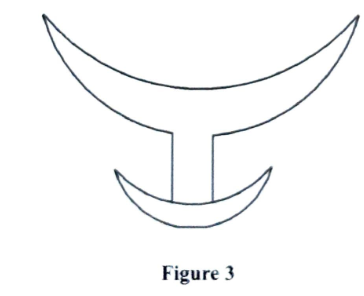Q6.KCSE Paper 1 2020 Physics

6. Figure 4 shows a uniform rod AB of length 1m and weigh 8N pivoted at 20cm from one end. It is balanced by supporting it with a string attached to a fixed support. The tension in the string is 4N. Determine the position of the string from end A

• Sum of clockwise moments = sum of anti-clockwise moments
• 4 x  x   =  8 x 30
• 4x = 240
•  x=60cm
• Position of string = 60 + 20 =80cm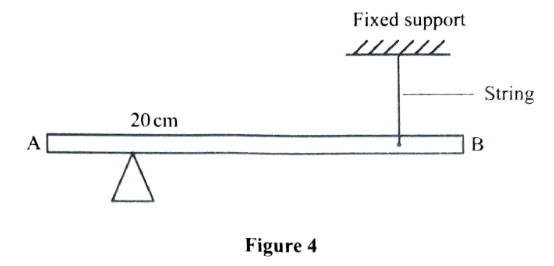Q7.KCSE Physics Paper 1 2020 Q 7-9

7. Define cohesive forces

Forces of attraction between molecules of the same type

8. Two similar containers A and B are filled with equal masses of water at the same temperature. Container A is made up of copper while B is made up of glass. Heat is then supplied to the containers at the same rate. State with a reason the container in which water boils first                                                                            2mks

Container A. It is a better conductor of heat.

9. Figure 5 show a roof of a house over which wind is blowing. It was observed that when the speed of wind is increased, the roof is blown off. Explain this observation                                 2mks

• Due to the shape the wind at the top moves at a higher speed creating a region of low pressure (Bernoulli’s effect) at the top. The pressure difference between the top and inside produces an upward force causing the roof to be blown off.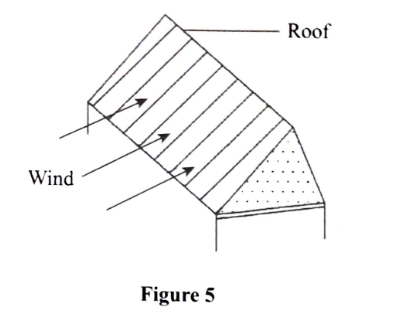Q8.KCSE 2020 Physics Paper 1

10.Figure 6 shows the velocity time graph of the motion of a stone thrown vertically upwards. From the graph determine the height reached by the stone                                                                                      3mks

Displacement = Area under the graph (the curve)

=  ½  x 2 x 20  =20m

Alternatively;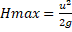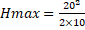= 20m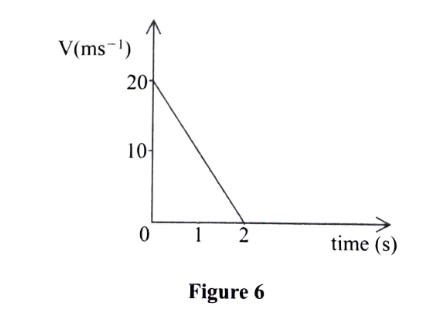Q9.KCSE 2020 Physics 1 Qs. 11-12

11. It is observed that when methylated spirit is poured on the palm, the palm feels colder as it dries up. Explain this observation.

• The spirit extracts latent heat of vaporization from the palm to evaporate. This causes cooling in the palm as it evaporates

12. Figure 7 shows a box on a weighing balance. The balanced is placed on the floor of a lift.

State what would be observed on reading the balance when ;

a. Lift is accelerating downwards

• Reads a smaller value than the weight of the box (weightlessness)

b. Lift moves downwards with a uniform velocity

• Reads the actual weight of the box

c. Lift is accelerating upwards

• Reads a bigger value than the weight of the box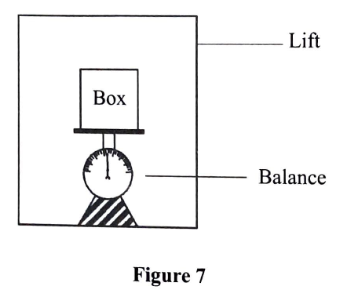Q10.KCSE Physics Paper 1  Q13-14

13. An object is placed on the surface of water in a beaker start to sink immediately. It is observed that it stopped sinking when half of its volume is below the water surface. State the reason for this observation

As it sinks upthrust increases, and stops when upthrust is equal to the weight of the object

Section B (55mks)

14. (a)  Figure 8 shows a bucket full of water and tied to one end of the string which is used to whirl it in a vertical circular path with a uniform speed V

i. State two forces acting on the bucket at any instant                                                                         2mks

Weight of the bucket

Tension on string

ii. Explain why tension is lowest at point A                                                                                         2mks

Part of the centripetal force required is provided by the weight. Tension and weight  act in the same direction therefore tension will be less

iii. The speed of the bucket is gradually reduced. Explain what is likely to be observed  when the bucket is at point A

Water is likely to pour out.

At certain minimum speed  the centripetal force is less than what is required to keep the motion of the bucket therefore some water spill out (T=0)

(b) A mass of 40kg is whirled at the end of a string in a circular horizontal at a speed of 12ms-1. (The stone and the string are in the same horizontal place). If the string is 1m long, determine the tension in the string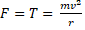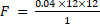=5.76N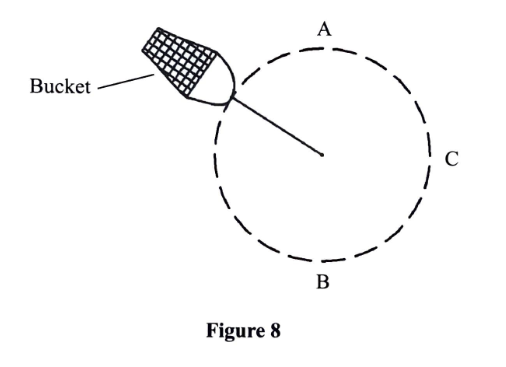Q11.KCSE 2020 Physics Paper 1  Q15(a)

15 (a)  Figure 9 shows a set-up used by a student to investigate how a siphon works.

(i) State what will be observed when the student sucks the tube at point A and release it                2mks

Upon sucking, the liquid flows in the delivery tube but stops on releasing because the sucking force is withdrawn

(ii) Explain the observation in a(i)                                                                                                   2mks

The liquid fails to flow on release because there is no pressure difference to push the liquid up the tube without sucking, the level of the container is above the liquid level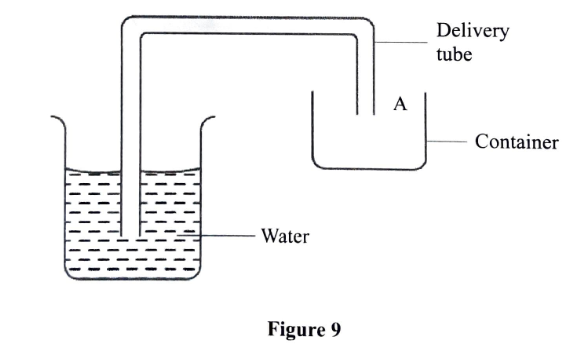Q12.F2 PHYSICS

Physics F2

1. An initial reading of a burette is 30.5 cm3. Three drops of water each of volume 0.6 cm3 are added. Determine the new reading of the burette.
2. A man of weight 800N exerts a pressure of 200,000Pa on the ground while standing on both feet. Calculate the area of each foot       (2 marks) How much pressure would he exert if he stands on one foot?   (2 marks)
3. Fifteen grams of common salt were added to 1000 cm3 of water. After all the salt had dissolved the volume of solution was found to be 998 cm3. Account for the decrease in volume of the solution.  (2 marks)
4. The stability of a body can be increased by increasing the base area and lowering its position of the centre of gravity. State one way of lowering its centre of gravity.(1 mark)
5. State the characteristics of images formed by a pinhole camera.   (2 marks)
6. Define the term “electromotive force” of a cell.                (1 mark)
7. State the principle of moments  (1mk)
8. A uniform metre rule balances at the 20cm mark when a 100g mass is hung at the 0cm mark.
9. Sketch a diagram of the balanced rule showing the two forces acting on it. (1 mark)
10. Calculate the weight of the rule.          (3 marks)
11. Calculate the reaction force (R) acting upwards on the system at the pivot. (2 marks)
12. State the basic law of magnetism        (1 mark)
13. The strength of a magnet cannot be measured beyond a certain point.   (2 marks)
14. The temperature increase weakens or destroys the magnetism of a magnet. (1 mark)
15. State two applications of iron as soft magnetic material.   (2 marks)
16. Define atmospheric pressure. (1 mark)
17. Explain why;
18. It is difficult to remove the lid from a preserving jar that was closed when the space above the food was full of steam.                (2 marks)
19. A force pump must be used instead of a lift pump to raise water from a deep well over 10m    (2 marks)
20. The barometric height at sea level is 76cm of mercury while that at a point on a highland is 74cm of mercury. What is the altitude of the point? Take g = 10N/kg, density of mercury = 13600 Kg/m3 and density of air as 1.25Kg/m3.                 (3 marks)
21. A current of 4.8A was passed through an electrolyte for ½ hours. Calculate the quantity of charge used.     (3 marks)
22. Recharging is one of the practices of maintenance of accumulators. State two measurements, which need to be taken to help you decide when an accumulator is due for charging.      (2 marks)